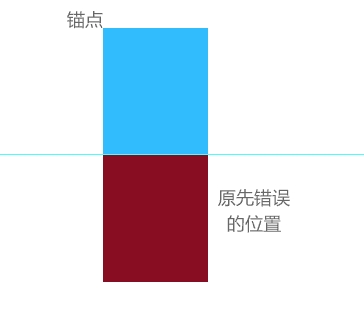1.cocos中的坐标系相当与数学中的坐标系第一象限。 前面对于方块精灵定位存在问题，用下面这个张图来示意。 我们设置了方块精灵的锚点在左上角，但是，前面放置网格点精灵都放在了深红色的位置了。这个会导致缩放的时候位置调整的计算出错的。 所以，先设定方块精灵的宽高，在从左上角锚点开始一个个防止网格点精灵。2.积分计数板就比较简单了，使用了一个label标签来显示，在手指离开屏幕时进行积分的累积判断。

3.缩放底部精灵也是简单的，只是位置计算的时候要注意点。缩放精灵，只需要将精灵的scale设置为要缩放的尺寸就可以了

``````/*创建底部三个可供拖动的精灵*/

createBottom : function(){

var y_offset = (cc.winSize.height - PublicData.item_height * PublicData.gridview_row) / 2;

for(var i = 0 ; i < 3 ; i++){

var type = Math.floor(Math.random() * PublicData.dialmond_type) + 1;

var sprite = new DiamondSprite(type);

sprite.attr({

x : (cc.winSize.width / 3 * i) + (cc.winSize.width / 3 - sprite.width * 0.8) / 2,

y : y_offset / 2 - sprite.height / 2 - sprite.height * 0.1,

});

sprite.scale = 0.8;//缩放精灵

this.array_bottom.push(sprite);

}

},
``````

4.清理满行，这里只需要逐行检测网格背景的状态数组，是否存在满行状态都为1的。 这里还是使用了动画，cocos中使用动画就是runAction(动作对象) cc.sequence()是一个可以同步执行的控制方法，它接受多个参数。例如：我们要执行完动画在执行一个回调方法时，这个函数是非常有用的。

``````/*清理满行*/
clearLine : function(){
for(var i = 0;i < PublicData.gridview_row ; ++i) {
var tag = true;

for(var j = 0; j < PublicData.gridview_col ; ++j){
if(this.map[i][j] != 1 ){
tag = false;
break;
}
}

if(tag){
for(var j = 0; j < PublicData.gridview_col ; ++j){
var self = this;
this.map_sprite[i][j].runAction(cc.sequence(cc.delayTime(0.005*j),cc.scaleTo(0,0.1),cc.callFunc(self.clearAction,self,[i,j])));
this.map[i][j] = 0 ;
}
}
}
},
``````

5.游戏是否结束检测，这里就要使用我们对方块精灵设置的数据结构，来进行逐个与背景网格相比较。

``````/*游戏结束检测*/
checkGameOver : function(){

for(var i = 0;i < PublicData.gridview_row ; ++i) {
for(var j = 0; j < PublicData.gridview_col ; ++j){
if(this.map[i][j] == 1 ){
continue;
}

for(var k = 0; k < this.array_bottom.length ; ++ k){
var list = this.array_bottom[k].vector;
var tag = 1;
for(var l = 0 ; l < list.length ; ++l){
var row = i + list[l];
var col = j + list[l];

if(row < 0 || row >= PublicData.gridview_row){
tag = 3;
break;
}
if(col < 0 || col >= PublicData.gridview_col){
tag = 3;
break;
}

if(this.map[row][col] == 1){
tag = 2;
break;
}
}
if(tag == 1) return true;
}
}
}

return false;
}
``````